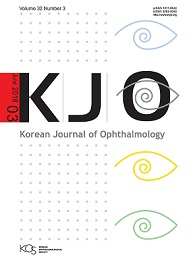상세검색
최근 검색어 전체 삭제
다국어입력
즐겨찾기0KCI등재 학술저널

# Choroidal Blood Flow Change in Eyes with High Myopia

• 등재여부 : KCI등재
• 2015.10
• 309 - 314 (6 pages)

Purpose: To evaluate choroidal blood flow changes in eyes with high myopia according to the pulsatile components of ocular blood flow analysis. Methods: A total of 104 subjects (52 males and 52 females) were included in this study. One eye of each participant was randomly selected and assigned to one of four refractive groups, designated as, hyperopes (n = 20; refractive error, ≥+1.00 diopter [D]), emmetropes (n = 28; refractive error, ±0.75 D), lower myopes (n = 33; refractive error, -1.00 to -4.75 D), and high myopes (n = 23; refractive error, ≤-5.00 D). Components of pulse amplitude (OBFa), pulse volume (OBFv), pulse rate (OBFr), and pulsatile ocular blood flow (POBF) were analyzed using a blood flow analyzer. Intraocular pressure and axial length were measured. Results: Pulsatile components of OBFa, OBFv, and POBF showed positive correlations with refractive error and showed negative correlations with axial length (r = 0.729, r = 0.772, r = 0.781, respectively, all p < 0.001; r= -0.727, r = -0.762, r = -0.771, respectively, all p < 0.001). The correlations of refractive error and axial length with OBFr were irrelevant (r = -0.157, p = 0.113; r = 0.123, p = 0.213). High myopes showed significantly lower OBFa, OBFv, and POBF than the other groups (all p < 0.001). Conclusions: Axial length changes in high myopes potentially influence choroidal blood flow, assuming the changes are caused by narrowing of the choroidal vessel diameter and increasing rigidity of the choroidal vessel wall. These finding explains the influence of axial length on OBFa, OBFv, and POBF, but not on OBFr. Thus, changes in axial length and the possible influence of these changes on the physical properties of choroidal vessels is the mechanism believed to be responsible for putting high myopes at risk for ocular vascular diseases.

Materials and Methods

Results

Discussion

Conflict of Interest

Acknowledgements

References

로딩중